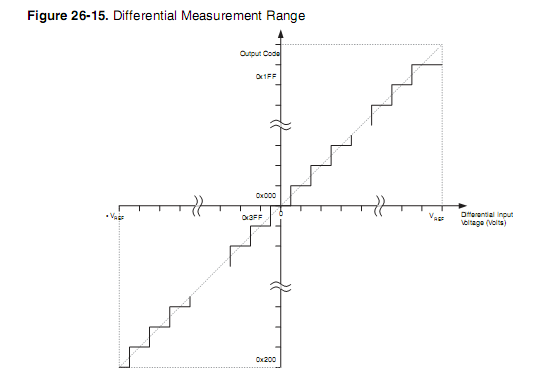### [emonLib] Why is it 1023 in "double I_RATIO = ICAL *((SUPPLYVOLTAGE/1000.0) / 1023.0);" ?

Hi.

There seems to be a debate on whether the value used in readVcc should be 1126400 or 1125300. I found both on the internet. See here, for instance.

This value is supposed to be 1000 * 1.1 * 1023 (or 1024). Where 1.1 is the reference voltage used.

The max value the ADC can deliver is 1023. But this is just a range.

The spec of the AtMega says ADC = Vin * 1024 / Vref, which suggests 1024 should be used.

The value used in emonLib is 1126400. The last guy talking on the page I mentioned says otherwise.

My understanding is 1024 is the correct choice, but I guess if it was obvious, everyone would agree on that one.

Besides, I don't understand the 1023 in

double I_RATIO = ICAL *((SUPPLYVOLTAGE/1000.0) / 1023.0);

Shouldn't it be 1024 for the same reason ?

### Re: [emonLib] Why is it 1023 in "double I_RATIO = ICAL *((SUPPLYVOLTAGE/1000.0) / 1023.0);" ?

My understanding of it is that there are 1024 'steps', so  0 to 1023 is 1024 steps.  In this case 1023 would be correct.

Of course, I've been wrong before....

Dennis

### Re: [emonLib] Why is it 1023 in "double I_RATIO = ICAL *((SUPPLYVOLTAGE/1000.0) / 1023.0);" ?

I think it should be 1024. The Atmel document http://www.atmel.com/Images/doc2559.pdf throws a little more light on the subject, and the diagram Fig. 26-15 on Page 288 of Atmels doc2549.pdf pretty much settles the matter, albeit for a +/- 9-bit input, by showing that the last step is 1½ LSB wide.Pedantic arguments aside, in practice I think it makes no difference because it is going to take a seriously expensive meter to tell the difference with any degree of certainty, even on a measurement of direct voltage. Especially when the gain error is +/- 2 LSB.

### Re: [emonLib] Why is it 1023 in "double I_RATIO = ICAL *((SUPPLYVOLTAGE/1000.0) / 1023.0);" ?

Ahh, i muddled myself and meant 1024 would be correct, with the 0 being 1st step, 1023 being 1024.

### Re: [emonLib] Why is it 1023 in "double I_RATIO = ICAL *((SUPPLYVOLTAGE/1000.0) / 1023.0);" ?

"Ahh, i muddled myself and meant 1024 would be correct, with the 0 being 1st step, 1023 being 1024."

And I thought you'd got it wrong for a totally obscure reason (the 1½ LSB last step).  When you look at that diagram, and think about "nearest value", it all makes sense and the last step being different is the unavoidable consequence.

### Re: [emonLib] Why is it 1023 in "double I_RATIO = ICAL *((SUPPLYVOLTAGE/1000.0) / 1023.0);" ?

Hi.

I think both the diagram and the formula (ADC = Vin * 1024 / Vref) state clearly that 1024 is the correct value.

This said, I still don't get the 1023 in

`double I_RATIO = ICAL *((SUPPLYVOLTAGE/1000.0) / 1023.0);`

First, in readVcc, the ADC is used to read the (sharp) 1,1 V voltage, to calibrate the Vcc reference :

ADC = Vin * 1024 / Vref, where Vin = 1,1 V and Vref = Vcc

in other words

Vref = Vin * 1024 / ADC
Vcc(mV) = 1.1 * 1024 * 1000 / ADC = 1126400 / ADC

Then, the precise Vcc measure is used to rectify the measurement from analogRead :

ADC = Vin * 1024 / Vref, where Vin is what we want to measure and Vref = Vcc
Vin = ADC * Vcc / 1024

which yields
Vin = ADC * (Vcc(mV) / 1000) / 1024

By the way, I totally agree with Robert : I don't need this kind of precision at all. I'm just trying to understand the code.

### Re: [emonLib] Why is it 1023 in "double I_RATIO = ICAL *((SUPPLYVOLTAGE/1000.0) / 1023.0);" ?

My guess would be the '1023' comes from the same misunderstanding that was argued in your link. I think we ought to ask Glyn.

### Re: [emonLib] Why is it 1023 in "double I_RATIO = ICAL *((SUPPLYVOLTAGE/1000.0) / 1023.0);" ?

I could initiate a pull request with a patch for this, to raise attention. But it's a bit of an overkill considering the triviality of the (potential) change. Besides, it is not that important.

I changed the topic title to be more explicit.

### Re: [emonLib] Why is it 1023 in "double I_RATIO = ICAL *((SUPPLYVOLTAGE/1000.0) / 1023.0);" ?

I don't get where you got the Vin = 1.1 mV.

### Re: [emonLib] Why is it 1023 in "double I_RATIO = ICAL *((SUPPLYVOLTAGE/1000.0) / 1023.0);" ?

It is not 1.1 mV. It is the internal reference 1.1 V. See the Atmel data sheet.